# Selina Concise Chemistry Solution for ICSE Class 10 Chapter 8 Study of Compounds – Hydrogen Chloride

In class 10 Selina chemistry textbook, chapter 8 basically deals with understanding the preparation of hydrogen chloride from certain compounds. Students also learn about the fountain experiment to determine the density and solubility of hydrogen chloride, acidic properties of hydrogen chloride solution as well as it’s reaction with ammonia. Notably, while dealing with this chapter students have to also conduct experiments to prove a certain hypothesis. To help students get clarity on all the important concepts and find correct answers to all the questions given in the chapter, we are providing free concise chemistry class 10 ICSE solutions for chapter 8 here.

## Download PDF Of Selina Concise Chemistry Solution for ICSE Class 10 Chapter 8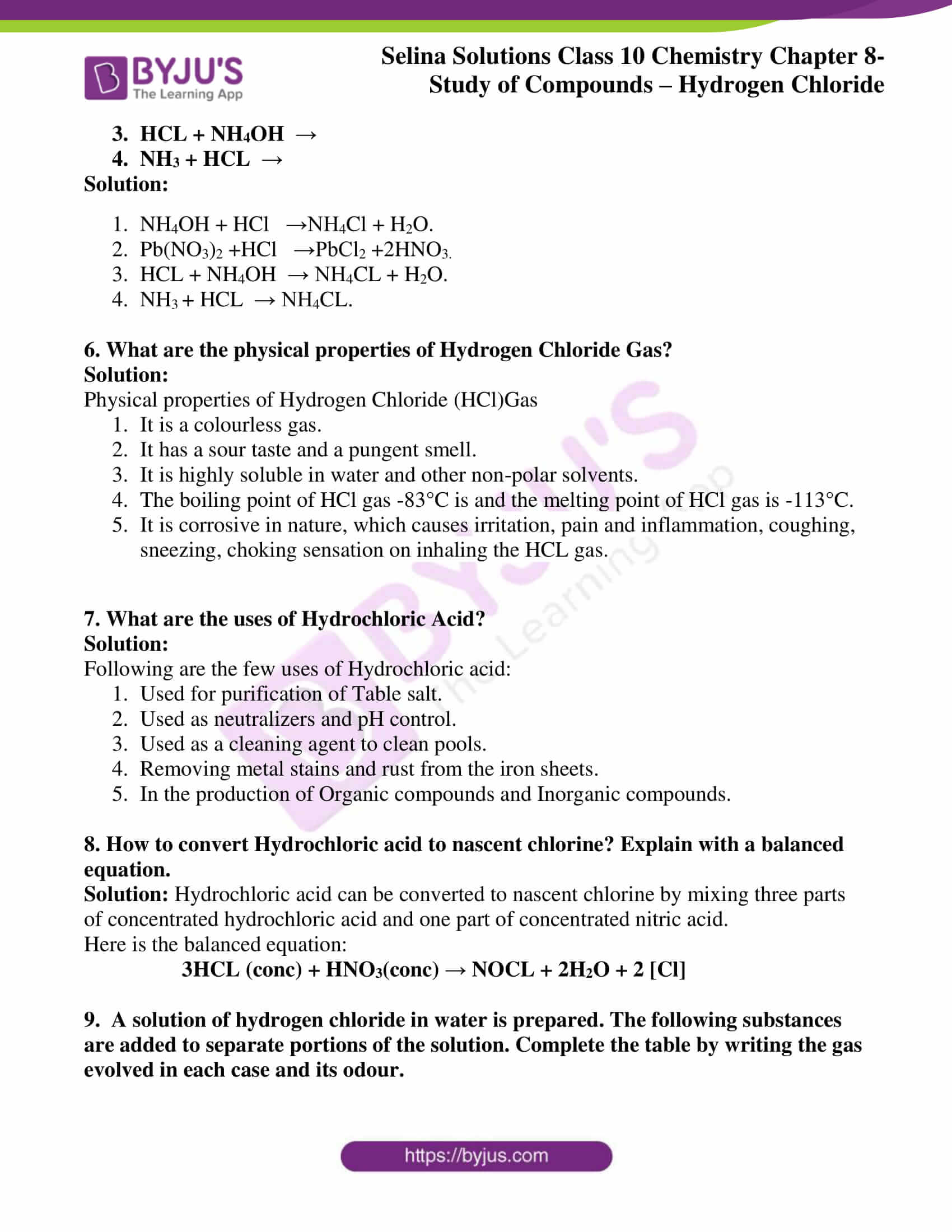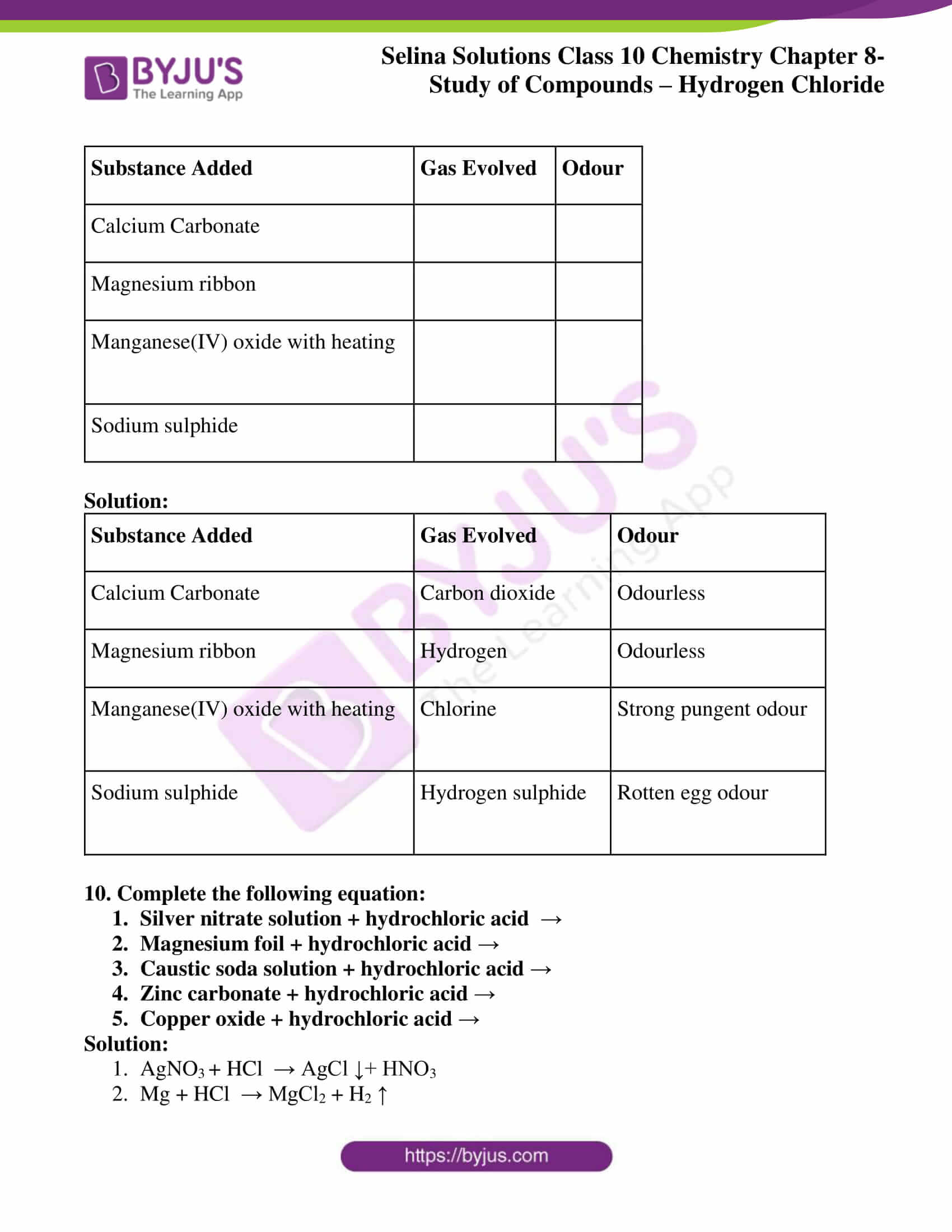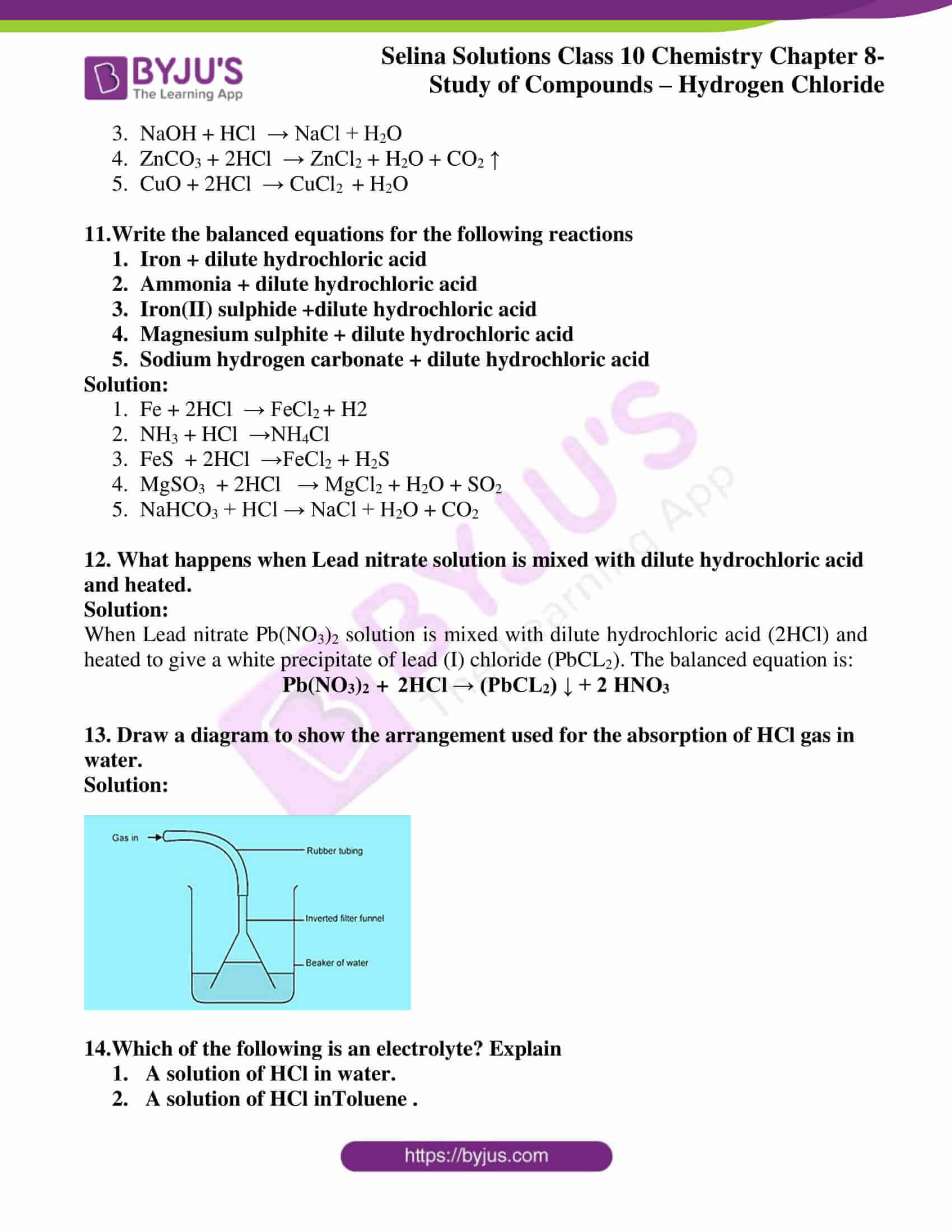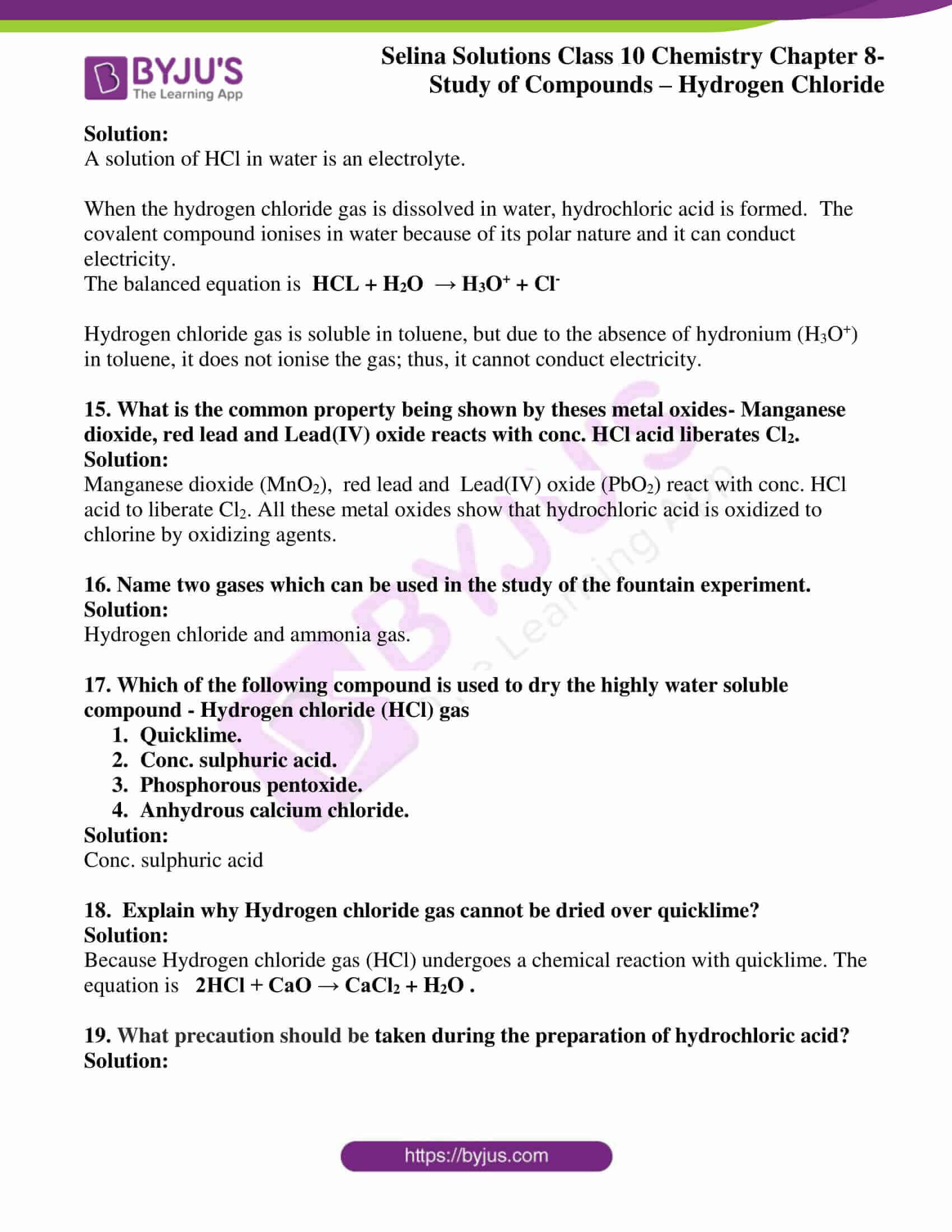### Access Selina Concise Chemistry Solution for ICSE Class 10 Chapter 8

Q1. Which acid used in the preparations of Hydrogen Chloride gas?

Solution:

Concentrated Sulfuric acid (H2SO4)

Q2. The dilute hydrochloric acid cannot be concentrated by boiling beyond 22.2 per cent. Why?

Solution:

Because the molecules of dilute hydrochloric acid HCl(g) get mixed with water vapour.

Q3. Give examples of two colourless gases, which combine to produce a white solid.

Solution:

Hydrogen chloride and ammonia.

Q4. How will you prove that hydrochloric acid contains hydrogen chlorine?

Solution:

We can prove that hydrochloric acid contains both hydrogen and chlorine by the following experiment.

Step-1

Take a voltameter used for electrolysis of water, fitted with the platinum cathode and a graphite anode.

Step-2

Pour 4 molar HCl into the voltameter and pass direct current.

Step-3

Keep the apparatus undisturbed and wait for the reaction to occur. The colourless gas is evolved at the cathode and a greenish gas is evolved at the anode.

Step-4

Now bring the burning splinter closer to the colourless gas, it bursts into a flame indicating the evolved gas is hydrogen gas.

Step-5

Place the moist starch iodide paper towards the greenish-yellow gas, the gas turns blue-black, indicating the evolved gas is chlorine.

Therefore, this experiment proves that hydrochloric acid contains both hydrogen and chlorine. The equation for this experiment is

2HCl – >500°c→   H2 + Cl2

Q 5. Complete and balance the following reactions:

1. NH4OH + HCl   →
2. Pb(NO3)2 +HCl   →
3. HCL + NH4OH  →
4. NH3 + HCL  →

Solution:

1. NH4OH + HCl   →NH4Cl + H2O.
2. Pb(NO3)2 +HCl   →PbCl2 +2HNO3.
3. HCL + NH4OH  → NH4CL + H2O.
4. NH3 + HCL  → NH4CL.

Q6. What are the physical properties of Hydrogen Chloride Gas?

Solution:

Physical properties of Hydrogen Chloride (HCl)Gas

1. It is a colourless gas.
2. It has a sour taste and a pungent smell.
3. It is highly soluble in water and other non-polar solvents.
4. The boiling point of HCl gas -83°C is and the melting point of HCl gas is -113°C.
5. It is corrosive in nature, which causes irritation, pain and inflammation, coughing, sneezing, choking sensation on inhaling the HCL gas.

Q7. What are the uses of Hydrochloric Acid?

Solution:

Following are the few uses of Hydrochloric acid:

1. Used for purification of Table salt.
2. Used as neutralizers and pH control.
3. Used as a cleaning agent to clean pools.
4. Removing metal stains and rust from the iron sheets.
5. In the production of Organic compounds and Inorganic compounds.

Q8. How to convert Hydrochloric acid to nascent chlorine? Explain with a balanced equation.

Solution: Hydrochloric acid can be converted to nascent chlorine by mixing three parts of concentrated hydrochloric acid and one part of concentrated nitric acid.

Here is the balanced equation:

3HCL (conc) + HNO3(conc) NOCL + 2H2O + 2 [Cl]

Q9.  A solution of hydrogen chloride in water is prepared. The following substances are added to separate portions of the solution. Complete the table by writing the gas evolved in each case and its odour.

 Substance Added Gas Evolved Odour Calcium Carbonate Magnesium ribbon Manganese(IV) oxide with heating Sodium sulphide

Solution:

 Substance Added Gas Evolved Odour Calcium Carbonate Carbon dioxide Odourless Magnesium ribbon Hydrogen Odourless Manganese(IV) oxide with heating Chlorine Strong pungent odour Sodium sulphide Hydrogen sulphide Rotten egg odour

Q10. Complete the following equation:

1. Silver nitrate solution + hydrochloric acid
2. Magnesium foil + hydrochloric acid
3. Caustic soda solution + hydrochloric acid
4. Zinc carbonate + hydrochloric acid
5. Copper oxide + hydrochloric acid

Solution:

1. AgNO3 + HCl  → AgCl ↓+ HNO3
2. Mg + HCl  → MgCl2 + H2
3. NaOH + HCl  → NaCl + H2O
4. ZnCO3 + 2HCl  → ZnCl2 + H2O + CO2
5. CuO + 2HCl  → CuCl2  + H2O

Q11. Write the balanced equations for the following reactions

1. Iron + dilute hydrochloric acid
2. Ammonia + dilute hydrochloric acid
3. Iron(II) sulphide +dilute hydrochloric acid
4. Magnesium sulphite + dilute hydrochloric acid
5. Sodium hydrogen carbonate + dilute hydrochloric acid

Solution:

1. Fe + 2HCl  → FeCl2 + H2
2. NH3 + HCl  →NH4Cl
3. FeS  + 2HCl  →FeCl2 + H2S
4. MgSO3  + 2HCl   → MgCl2 + H2O + SO2
5. NaHCO3 + HCl → NaCl + H2O + CO2

Q12. What happens when Lead nitrate solution is mixed with dilute hydrochloric acid and heated.

Solution:

When Lead nitrate Pb(NO3)2 solution is mixed with dilute hydrochloric acid (2HCl) and heated to give a white precipitate of lead (I) chloride (PbCL2). The balanced equation is:

Pb(NO3)+  2HCl → (PbCL2) ↓ + 2 HNO3

Q13. Draw a diagram to show the arrangement used for the absorption of HCl gas in water.

Solution: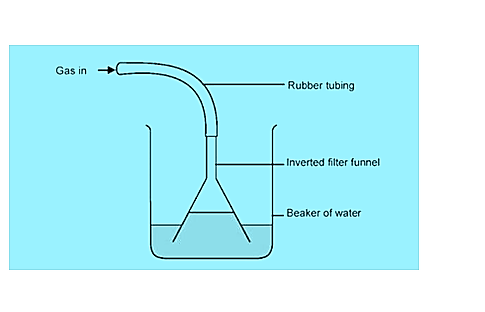14. Which of the following is an electrolyte? Explain.

1.  A solution of HCl in water.
2.  A solution of HCl inToluene .

Solution:

A solution of HCl in water is an electrolyte.

When the hydrogen chloride gas is dissolved in water, hydrochloric acid is formed.  The covalent compound ionises in water because of its polar nature and it can conduct electricity.

The balanced equation is  HCL + H2O  → H3O+ + Cl

Hydrogen chloride gas is soluble in toluene, but due to the absence of hydronium (H3O+) in toluene, it does not ionise the gas; thus, it cannot conduct electricity.

15. What is the common property being shown by theses metal oxides- Manganese dioxide, red lead and Lead(IV) oxide reacts with conc. HCl acid liberates Cl2.

Solution:

Manganese dioxide (MnO2),  red lead and  Lead(IV) oxide (PbO2) react with conc. HCl acid to liberate Cl2. All these metal oxides show that hydrochloric acid is oxidized to chlorine by oxidizing agents.

16. Name two gases which can be used in the study of the fountain experiment.

Solution:

Hydrogen chloride and ammonia gas.

17. Which of the following compound is used to dry the highly water-soluble compound – Hydrogen chloride (HCl) gas.

1. Quicklime.
2. Conc. sulphuric acid.
3. Phosphorous pentoxide.
4. Anhydrous calcium chloride.

Solution:

Conc. sulphuric acid.

18.  Explain why Hydrogen chloride gas cannot be dried over quicklime?

Solution:

Because Hydrogen chloride gas (HCl) undergoes a chemical reaction with quicklime.

The equation is   2HCl + CaO → CaCl2 + H2O .

19. What precaution should be taken during the preparation of hydrochloric acid?

Solution:

Diluting acid means adding water to the concentrated acid, therefore a proper safety precaution should be taken before starting the procedure. Here is the safety precaution followed in the laboratory.

• Protective gloves and a chemical-resistant apron should be worn.
• The delivery tube should be dipped in drying agent conc. H2SO4.
• The lower end of the thistle funnel must be dipped in conc. Sulphuric acid.
• Always, make sure the temperature should be maintained at nearly 200oC.

20.  State your observation for the following reactions.

1. Dilute HCl is added to sodium carbonate crystals.
2. Copper sulphide is treated with dilute hydrochloric acid.
3. A few drops of dil. HCl is added to silver nitrate solution, followed by addition of NH4OH solution.

Solution:

1. When sodium carbonate crystals are added to dil HCl, sodium chloride is formed along with the water and carbon dioxide gas. A brisk effervescence is evolved This is called the neutralisation reaction. The chemical equation for this reaction is as follows:

Na2CO3 + 2 HCL  → 2 NaCl + H2O + CO2

2. When copper sulphide is treated with dilute hydrochloric acid, black coloured copper sulphite turns to blue colour by evolving hydrogen sulphide gas with the rotten egg odour. The chemical equation for this reaction is as follows:

CuS + HCl  → CuCl2 + H2

3. When a  few drops of dil. HCl is added to silver nitrate solution, followed by addition of NH4OH solution, a curdy white precipitate of silver chloride is formed which is soluble in ammonium hydroxide. The chemical equation for this reaction is as follows:

AgNO3  + HCl → AgCl + HNO3

The solutions have been prepared by our experts and they act a very useful study tool for students. Once they get acquainted with the solutions, students will get insight into all the important topics and questions that they should focus on before the exams. With these Selina textbook solutions, students can not only prepare well but score higher marks in the exams.

The given solutions are as per the 2019-20 Concise Selina textbook. The Selina Solutions for the academic year 2023-24 will be updated soon.